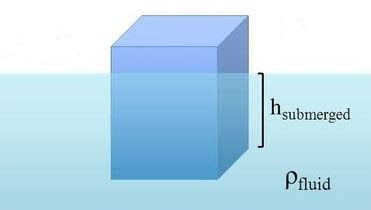Cody

# Problem 44292. Archimedes principle

According to Archimedes principle, the buoyancy force is equal to the weight of fluid displaced by the submerged portion of an object. For the block depicted in the figure, determine the height h of the portion that is above the fluid. Employ the following values for your computation: 1m x 1m x 0.7m are the total dimensions of the block, rho=density of the block=583 kg/m^3, and x=density of fluid=1020 kg/m^3. Note that the fluid is variable, so the density (x) value may change.### Solution Stats

63.64% Correct | 36.36% Incorrect
Last Solution submitted on May 13, 2020